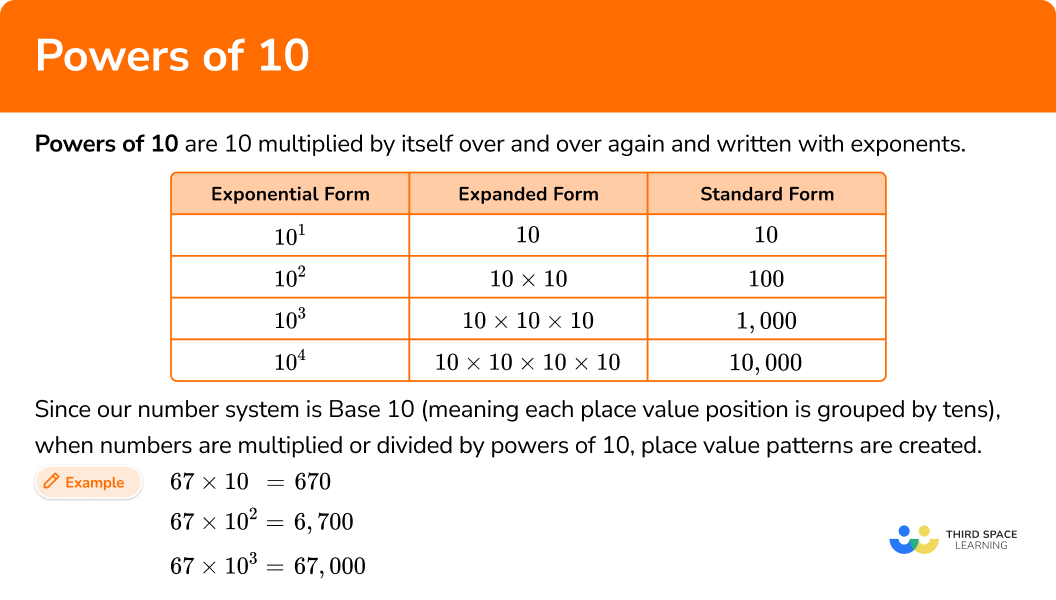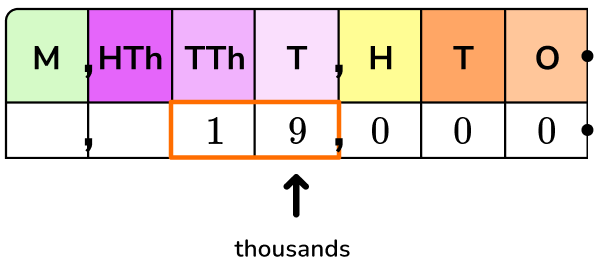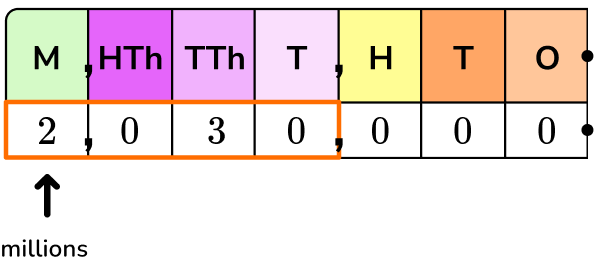# Powers of 10

Here you will learn about powers of 10, including what they are and how to write and solve equations using powers of 10.

Students will first learn about powers of 10 as part of numbers and operations in base ten in 5th grade.

## What are powers of 10?

Powers of \bf{10} are 10 multiplied by itself over and over again and written with exponents.

For example,

Exponential FormExpanded FormStandard Form
10^1 10 10
10^2 10 \times 10 100
10^3 10 \times 10 \times 10 1,000
10^4 10 \times 10 \times 10 \times 10 10,000

Since our number system is Base 10 (meaning each place value position is grouped by tens), when numbers are multiplied or divided by powers of 10, place value patterns are created.

For example,

\begin{aligned} & 67 \times 10=670 \\\\ & 67 \times 10^2=6,700 \\\\ & 67 \times 10^3=67,000 \end{aligned}

The digits from the original number (67) are always in the product, but they increase in place value.

Notice that each time an additional 10 is multiplied, the number of place value positions the digits move increases by one.

This is also true when dividing with powers of 10.

For example,

\begin{aligned} & 67 \div 10=6.7 \\\\ & 67 \div 10^2=0.67 \\\\ & 67 \div 10^3=0.067 \end{aligned}

The digits for the original number (67) are always in the quotient, but they decrease in place value.

Notice that each time an additional 10 is divided, the number of places the digits move increases by one.

### What are powers of 10?## Common Core State Standards

How does this relate to 5th and 6th grade math?

• Grade 5 – Numbers and Operations in Base Ten (5.NBT.2)
Explain patterns in the number of zeros of the product when multiplying a number by powers of 10, and explain patterns in the placement of the decimal point when a decimal is multiplied or divided by a power of 10. Use whole-number powers of 10 to denote powers of 10.

## How to use powers of 10

In order to represent a number as a power of 10 :

1. Show the number as an expression with multipliers of \bf{10} .
2. Count the number of \bf{10} s in the expression to create the power of \bf{10} .
3. Write the equation.

In order to solve an expression with a power of 10 :

1. Use place value reasoning to identify how the power of \bf{10} will change the number.
2. Shift the digits left if multiplying and shift the digits right if dividing.

## Powers of 10 examples

### Example 1: number as a power of 10

Write 45,000 as a power of 10.

1. Show the number as an expression with multipliers of \bf{10} .

45,000 is the same as 45 thousands or 45 \times 1,000.

1,000 as a product of tens is 10 \times 10 \times 10, so…

45,000=45 \times 10 \times 10 \times 10

2Count the number of \bf{10} s in the expression to create the power of \bf{10} .

45,000=45 \times 10 \times 10 \times 10

There are 3 tens being multiplied. Use 10 as the base and 3 as the exponent.

3Write the equation.

45,000=45 \times 10^3

*Note: You can show 45,000 with other powers of 10. See how in the next example.

### Example 2: number as multiple powers of 10

Write 1,030,000 as two different powers of 10.

Show the number as an expression with multipliers of \bf{10} .

Count the number of \bf{10} s in the expression to create the power of \bf{10} .

Write the equation.

### Example 3: expression with a power of 10 – product

Solve 5.3 \times 10^6 using place value reasoning.

Use place value reasoning to identify how the power of \bf{10} will change the number.

Shift the digits left if multiplying and shift the digits right if dividing.

### Example 4: expression with a power of 10 – quotient

Solve 80,800,000 \div 10^7 using place value reasoning.

Use place value reasoning to identify how the power of \bf{10} will change the number.

Shift the digits left if multiplying and shift the digits right if dividing.

### Example 5: expression with a power of 10 – missing operation and power of 10

Complete the equation using an operation with a power of 10 :

Use place value reasoning to identify how the power of \bf{10} will change the number.

Shift the digits left if multiplying and shift the digits right if dividing.

### Example 6: expression with a power of 10 – missing dividend

Complete the equation:

Use place value reasoning to identify how the power of \bf{10} will change the number.

Shift the digits left if multiplying and shift the digits right if dividing.

### Teaching tips for powers of 10

• Throughout teaching this skill, never forget that the main purpose is for students to explain patterns and deepen their understanding of place value. While the rules “moving the decimal point” or “adding zeros” may come up in discussions, students who memorize these rules and never make place value connections will not truly master the standard – even if they can rotely solve these types of problems.

• Worksheets are useful for this skill, but be sure they include a variety of question types. Worksheets that only include solving for the product or quotient and/or do not ask students to explain the place value connection, will promote only a rote understanding of this skill.

### Easy mistakes to make

• Not knowing what to do when there are \bf{0} s within (not just at the end)
Students are quick to notice a pattern between the power of 10 and the amount of 0 s at the end of many numbers. However, if students only see numbers that have 0 s at the end, they may create solving strategies that don’t work with all numbers.

This could cause mistakes when dealing with numbers like 304,000 or expressions like 5.06 \times 10^4. It is important to expose students to these types of numbers and equations from the beginning, to help avoid this misconception.

• Using the “adding zeros” rule incorrectly
When multiplying whole numbers by powers of 10, students will notice that for each exponent a zero is being “added.” This is caused by the same pattern that “moves” the decimal. Students should use this rule only if they understand why it works. Otherwise it can lead to mistakes if applied to other types of equations.
For example,

• Multiplying the base and the exponent
The base number is always the only number being multiplied. The exponent just tells us how many times to multiply the base. It is incorrect to multiply the base by the exponent.
For example,

• Forgetting about an exponent of \bf{1}
10 by itself is technically 10^1. It is not typically written this way, but it is important to remember that the exponent of 1 is there.

### Practice powers of 10 questions

1. Which expression shows 19,000 as a power of 10?

19 \times 10^419 \times 10^219 \times 10^519 \times 10^319,000 is the same as 19 thousands or 19 \times 1,000.

1,000 as a product of tens is 10 \times 10 \times 10, so…

19,000=19 \times 10 \times 10 \times 10

There are 3 tens being multiplied. Use 10 as the base and 3 as the exponent.

19,000=19 \times 10^3

2. Which expression shows 2,030,000 as a power of 10?

20.3 \times 10^42.03 \times 10^620.3 \times 10^32.03 \times 10^82,300,000 is the same as 2.03 millions or 2.03 \times 1,000,000.

1,000,000 as a product of tens is 10 \times 10 \times 10 \times 10 \times 10 \times 10, so…

2,030,000=2.03 \times 10 \times 10 \times 10 \times 10 \times 10 \times 10

There are 6 tens being multiplied. Use 10 as the base and 6 as the exponent.

2,030,000=2.03 \times 10^6

3. Solve 180 \times 10^5.

180,0001,800,00018,000,00010,800,00010^5=10 \times 10 \times 10 \times 10 \times 10

Since each place value is 10 times larger than the position to the right, 180 will be 5 place value positions larger after it is multiplied by 10^5.

Multiplying by 10, moves all the digits up one position.

For \times 10^5, shift the digits 5 times to the left.Notice in this case, since 180 is a whole number, that \times 10^5 also “adds” 5 zeros.

This happens for the same reason the decimal point “moves.”

The place value is growing by 5 positions, so each new position needs a 0 as a place holder.

4. Solve 4,100 \div 10^4.

41,000,0004,100,0004.10.4110^4=10 \times 10 \times 10 \times 10

Since each place value is 10 times smaller than the position to the left, 4,100 will be 4 place value positions smaller after it is divided by 10^4.

Dividing by 10, shifts all the digits down one position.

For \div 10^4, shift the digits 4 times to the right.5. Which equation is true?

4.6 \times 10^8=460,000,0004.6 \times 10^4=460,0004.6 \div 10^8=460,000,0004.6 \div 10^4=460,000The 4 in 4.6 is in the ones position.

The 4 in 460,000,000 is in the hundred-millions position.

This is a change of 8 place value positions.

This means the power of 10 is 10^8.The place value of the 4 and 6 grows larger by 8 positions. Because of this, the digits shift to the left and the operation is multiplication.

6. Which number completes the equation?0.70270.270,200702,00010^3=10 \times 10 \times 10

Since each place value is 10 times smaller than the position to the left, 702 is 3 place value positions smaller than the dividend that was divided by 10^3.The 7, 0 , and 2 in dividend will be 3 place value positions larger than in the quotient, 702.

Even though the equation operation is division, to find the quotient, work backwards and shift the digits to the left.

702,000 \div 10^3 = 702

## Powers of 10 FAQs

How are powers of \bf{10} useful in mathematics?

For this standard, powers of 10 are useful in identifying and understanding place value patterns. In later grades, this skill is referred to as scientific notation and is used to represent very small numbers and very large numbers in a more efficient way.

What is the rule for powers of \bf{10} ?

The number of times the decimal place moves is the same as the exponent when 10 is the base. The operation, multiplication or division, tells which direction to move the decimal place.

Can there be a negative exponent in a power of \bf{10} ?

Yes, although this standard only covers positive powers, in later grades, students will work with negative powers of ten.

What decimal positions are used with powers of \bf{10} ?

Per the Common Core, in 5th grade, students work with the tenth, hundredth, and thousandth positions. However, in real world applications, powers of 10 have no limit as to how many decimal points they can represent. It is common that they include numbers up to billionth or even smaller positions. In fact, one of the purposes of powers of 10 is to make it easier to write very, very small numbers.

Can calculators be used to solve powers of \bf{10} ?

Yes, scientific calculators in particular include an exponent function that can be used to input and solve an equation with a power of 10.

## Still stuck?

At Third Space Learning, we specialize in helping teachers and school leaders to provide personalized math support for more of their students through high-quality, online one-on-one math tutoring delivered by subject experts.

Each week, our tutors support thousands of students who are at risk of not meeting their grade-level expectations, and help accelerate their progress and boost their confidence.